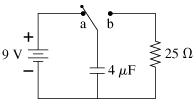# Problem: The switch in the figure has been in position a for a long time. It is changed to position b at t=0s.a) What is the charge Q on the capacitor immediately after the switch is closed?b) What the current I through the resistor immediately after the switch is closed?c) What is the charge Q on the capacitor at t=50 × 10-6 s?d) What is the current I through the resistor at t=50 × 10-6 s?e) What is the charge Q on the capacitor at t=200 × 10-6 s?f) What is the current I through the resistor at t=200 × 10-6 s?

###### FREE Expert Solution

The charge stored in a capacitor:

$\overline{){\mathbf{Q}}{\mathbf{=}}{\mathbf{C}}{\mathbf{V}}}$

Ohm's law:

$\overline{){\mathbf{V}}{\mathbf{=}}{\mathbf{I}}{\mathbf{R}}}$

Charge in capacitor after sometime:

$\overline{){\mathbf{Q}}{\mathbf{=}}{{\mathbf{Q}}}_{{\mathbf{0}}}{{\mathbf{e}}}^{\mathbf{\left(}\mathbf{-}\mathbf{t}\mathbf{/}\mathbf{R}\mathbf{C}\mathbf{\right)}}}$

(a)
Q = CV = 4 × 10-6 × 9 = 36 × 10-6 C
82% (106 ratings)##The switch in the figure has been in position a for a long time. It is changed to position b at t=0s.

a) What is the charge Q on the capacitor immediately after the switch is closed?

b) What the current I through the resistor immediately after the switch is closed?

c) What is the charge Q on the capacitor at t=50 × 10-6 s?

d) What is the current I through the resistor at t=50 × 10-6 s?

e) What is the charge Q on the capacitor at t=200 × 10-6 s?

f) What is the current I through the resistor at t=200 × 10-6 s?

Frequently Asked Questions

What scientific concept do you need to know in order to solve this problem?

Our tutors have indicated that to solve this problem you will need to apply the !! Resistor-Capacitor Circuits concept. If you need more !! Resistor-Capacitor Circuits practice, you can also practice !! Resistor-Capacitor Circuits practice problems.

What professor is this problem relevant for?

Based on our data, we think this problem is relevant for Professor Jamkhedkar's class at PDX.